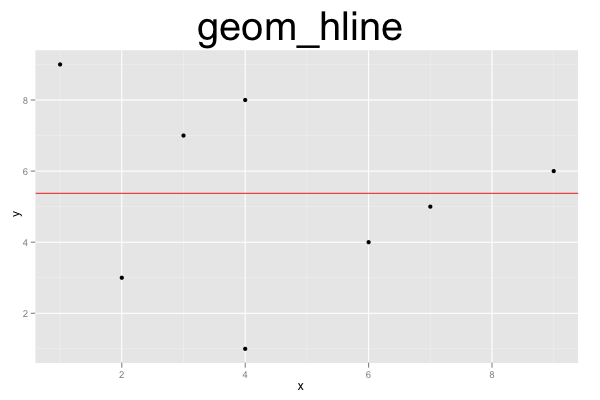## ggplot2 Quick Reference: geom_hline

A geom that draws a horizontal line defined by an y-axis intercept.

Default statistic: stat_hline

### Parameters

For geom_hline, whether or not one uses the default statistic (stat_hline) or the "do nothing" statistic (stat_identity), the available parameters and their meanings stay the same.

• yintercept - (required) intercept with the y axis of the horizontal line
• size - (default: 0.5) width of the line
• linetype - (default: 1=solid) the type of the line
• colour - (default: "black") the color of the line
• alpha - (default: 1=opaque) the transparency of the line

### Example

This plot consists of two layers. The bottom layer shows points, and the layer above it shows a red horizontal line. The line's yintercept is set to a constant value (which is the mean of the x values of all the points).```d=data.frame(x=c(1,2,3,4,4,6,7,9), y=c(9,3,7,1,8,4,5,6))
ggplot() +
geom_point(data=d, mapping=aes(x=x, y=y)) +
geom_hline(yintercept=mean(d\$y), color="red") +
opts(title="geom_hline", plot.title=theme_text(size=40, vjust=1.5))```